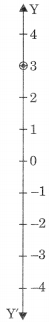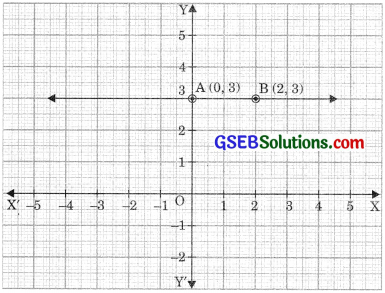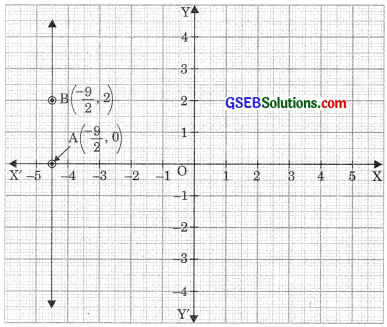# GSEB Solutions Class 9 Maths Chapter 4 Linear Equations in Two Variables Ex 4.4

Gujarat Board GSEB Solutions Class 9 Maths Chapter 4 Linear Equations in Two Variables Ex 4.4 Textbook Questions and Answers.

## Gujarat Board Textbook Solutions Class 9 Maths Chapter 4 Linear Equations in Two Variables Ex 4.4

Question 1.
Give the geometric representations of y = 3 as an equation
(i) in one variable.
(ii) in two variables
Solution:
The given equation is y = 3
(i) In one variable, y = 3
Representation of y = 3 on a number line
reflects a point. For this, we draw a vertical number line and locate the point.(ii) In two variables, y = 3 can be expressed as o.x + 1.y = 3This is a linear equation in two variables x and y which is represented by a line. All the values of x are permissible because 0.x is always 0. whereas y satisfies the relation y = 3. Therefore two solutions of the given equation are x = 0, y = 3 and x = 2, y = 3.
Hence the graph AB is a line parallel to x-axis at the distance of 3 units above the origin.Question 2.
Give the geometric representations of 2x + 9 = 0 as an equation
(i) in one variable (ii) in two variables
Solution:
The given equation is 2x + 9 = 0 on the number line is shown below.(ii) In one variable2x + 9 = 0
2x + 0.y + 9 = 0
This is a linear equation in two variables x andy which is represented by a line. Hence all the values of y are permissible because 0.y is always 0. Whereas x must satisfies the relation 2x + 9 = 0 i.e., x = $$\frac {-9}{2}$$
Hence two solutions of given equation are, x = $$\frac {-9}{2}$$ , y = 0, and x = $$\frac {-9}{2}$$ , y = 2
Hence AB is a line parallel to the y-axis and at a distance of $$\frac {9}{2}$$ units to the left of origin O.